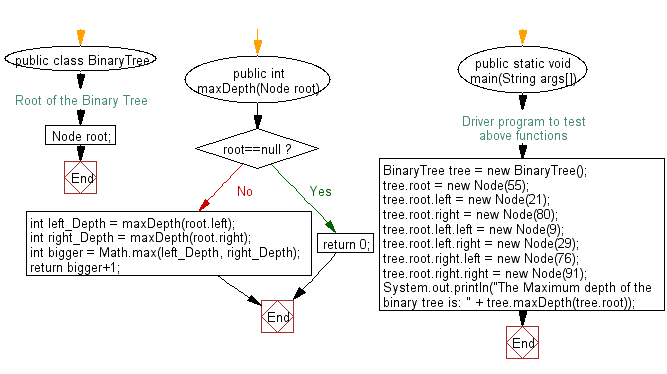﻿ Java: Find the maximum depth of a given a binary tree# Java Exercises: Find the maximum depth of a given a binary tree

## Java Basic: Exercise-130 with Solution

Write a Java program to find the maximum depth of a given a binary tree.
Sample Output: The Maximum depth of the binary tree is: 3

Sample Solution:

Java Code:

``````class Node
{
int data;
Node left, right;
public Node(int item)
{
data = item;
left = right = null;
}
}
public class BinaryTree
{
//Root of the Binary Tree
Node root;

public int maxDepth(Node root) {
if(root==null)
return 0;
int left_Depth = maxDepth(root.left);
int right_Depth = maxDepth(root.right);
int bigger = Math.max(left_Depth, right_Depth);
return bigger+1;
}
/* Driver program to test above functions */
public static void main(String args[])
{
BinaryTree tree = new BinaryTree();
tree.root = new Node(55);
tree.root.left = new Node(21);
tree.root.right = new Node(80);
tree.root.left.left = new Node(9);
tree.root.left.right = new Node(29);
tree.root.right.left = new Node(76);
tree.root.right.right = new Node(91);
System.out.println("The Maximum depth of the binary tree is: " + tree.maxDepth(tree.root));
}
}
```
```

Sample Output:

```The Maximum depth of the binary tree is: 3
```

Flowchart:Java Code Editor:

What is the difficulty level of this exercise?

Test your Programming skills with w3resource's quiz.

﻿

## Java: Tips of the Day

countOccurrences

Counts the occurrences of a value in an array.

Use Arrays.stream().filter().count() to count total number of values that equals the specified value.

```public static long countOccurrences(int[] numbers, int value) {
return Arrays.stream(numbers)
.filter(number -> number == value)
.count();
}
```

Ref: https://bit.ly/3kCAgLb